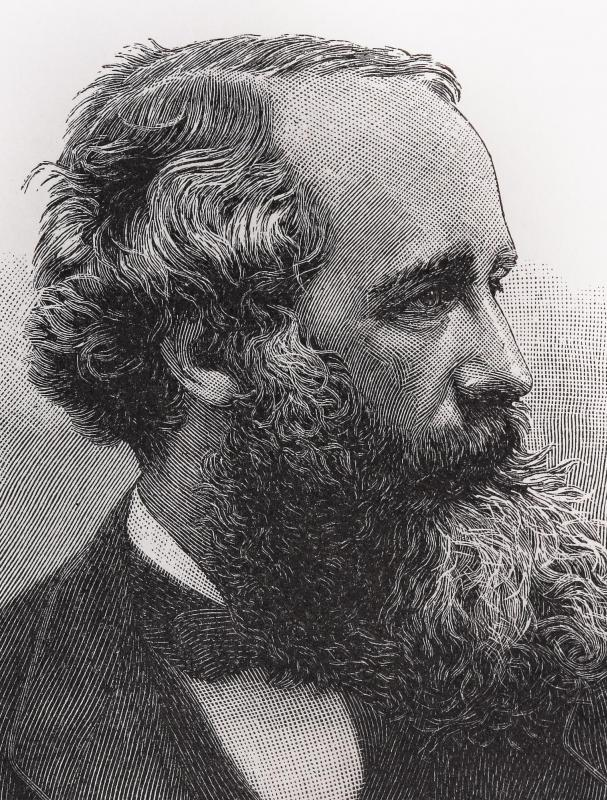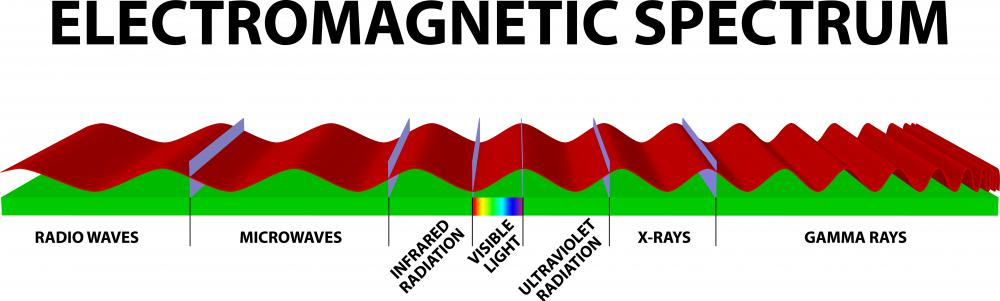# What Is Electromagnetic Theory?

David Isaac Rudel

Einstein’s theory of special relativity describes magnetism as the byproduct of electric force. Hence, these two forces can be considered different facets of a more fundamental force, which physicists call electromagnetism. Electromagnetic theory describes a collection of interconnected scientific claims used to answer questions about this force.Physicist James Clerk Maxwell was noted for his work with electromagnetism.

Physicists use fields as abstractions to describe how a system affects its surroundings. The electric field of a charged object represents the force that it would exert on a charged particle. The field is stronger closer to the object because electrostatic force decreases as the distance between two charges increases. Magnetic fields are similarly defined, except they describe the force exerted on a moving charged particle.

The most basic ideas in electromagnetic theory are “a changing electric field generates a magnetic field” and “a changing magnetic field generates an electric field.” These principles are quantified by Maxwell’s equations, named for James Clerk Maxwell, the Scottish physicist and mathematician whose work in the 19th century established the discipline by revolutionizing how physicists conceived of light. Maxwell’s equations also cast previously known relationships — Coulomb’s law and the Biot-Savart law — into the language of fields.

A charged particle generates a magnetic field as it moves, but the magnetic field is perpendicular to the motion of the particle. Furthermore, the effect this magnetic field has on a second moving charge is perpendicular to both the field and the motion of the second charge. These two facts cause even basic problems in electromagnetism to require complex, three-dimensional reasoning. Historically, the development of vectors in mathematics and science owes much of its progress to the work of physicists trying to abstract and simplify the use of electromagnetic theory.

In the 19th century, electromagnetic theory changed how physicists understood light. Newton had described light in terms of particles called corpuscles, but Maxwell claimed it was the manifestation of electric and magnetic fields that pushed each other through space. According to this conception, visible light, X-rays, radar, and many other phenomena are all inherently similar, each a combination of electric and magnetic fields varying at a different frequency. Scientists call the continuum of all such waves the electromagnetic spectrum.

The success of electromagnetic theory led to the collapse of the rest of Newtonian physics in the 20th century. Einstein realized that Maxwell’s theory required space and time to interdependent, different coordinates of a four-dimensional space-time. Moreover, Einstein’s theory of relativity showed that space was curved and the passage of time measured by one observer differed from that measured by another. These discoveries were all thoroughly incompatible with Newton’s theory of motion. Thus, the study of electromagnetism has, directly or indirectly, altered how physicists understand electricity, magnetism, light, space, time, and gravity.Visible light, X-rays, radar, are all inherently similar, and scientists call the continuum of all such waves the electromagnetic spectrum.

## You might also Like